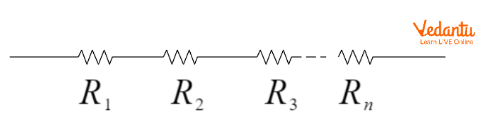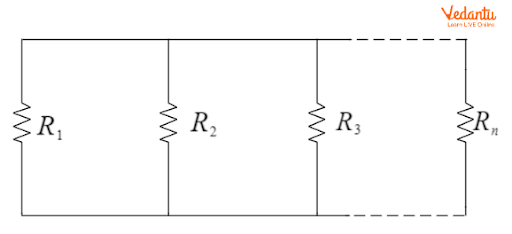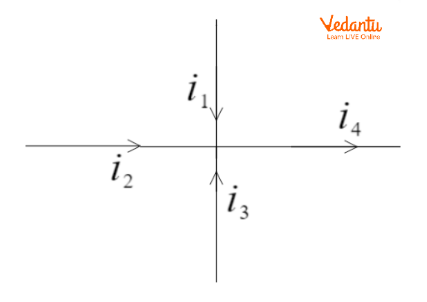Courses
Courses for Kids
Free study material
Offline Centres
More

# How to Solve Electrical Circuits - JEE Important TopicLast updated date: 03rd Dec 2023
Total views: 21.9k
Views today: 0.21k## What are Electrical Circuits?

An electric circuit consists of electrical components and provides a path for the current to flow. A basic electric circuit could consist of a voltage source, and a load that consumes the voltage or across which a potential drop is observed. When the circuit is closed, current flows through the circuit. Solving a basic electric circuit means to find the total resistance in the circuit, to determine the potential difference, or to find the current flowing in the circuit.

Several laws have been formulated to help solve a circuit. The most basic is Ohm’s law that is used to solve a simple circuit. As the circuit gets complex, i.e., several loops are present, then Kirchhoff’s Laws are used. The two laws of Kirchhoff are Kirchhoff’s Current Law (KCL) and Kirchhoff’s Voltage Law (KVL). We shall be discussing all of these in detail in this article.

## Finding Equivalent Resistances

The resistance or load is defined as the obstruction provided by several components of a circuit to the current flow. If there are two or more resistances present in the circuit, then it can be replaced with an equivalent resistance of the two resistances. The equivalent resistance can be determined using the following two methods.

1. When Resistances are Connected in Series:

The equivalent resistance for several resistances connected in series is equal to the sum of all individual resistances. Consider the below diagram.Resistances Connected in Series

The equivalent resistance (R) for the above circuit will be

$R={{R}_{1}}+{{R}_{2}}+{{R}_{3}}+...+{{R}_{n}}$

1. When Resistances are Connected in Parallel

The reciprocal of the equivalent resistance for several resistances connected in parallel is equal to the sum of reciprocals of all resistances in the circuit. Consider the below diagram.Resistances Connected in Parallel

The equivalent resistance (R) for the above circuit will be

$\frac{1}{R}=\frac{1}{{{R}_{1}}}+\frac{1}{{{R}_{2}}}+\frac{1}{{{R}_{3}}}+..+\frac{1}{{{R}_{n}}}$

## Ohm’s Law

This is the most fundamental law when dealing with circuits. It describes the relationship between voltage, current, and resistance. The statement of Ohm’s law is that ‘When the temperature and other physical quantities are constant, then the potential difference (V) across two terminals of a circuit is directly proportional to the current (I) flowing in the circuit.’Ohm’s Law

The constant of proportionality is the resistance in the circuit, denoted by ‘R’.

Thus, the statement of Ohm’s Law is formulated as $V= I~ \times ~R$

## Kirchhoff’s Current Law

Kirchhoff's Current law states “Algebraic sum of all electric currents arriving at a junction (also termed as node) is equal to zero”.

Suppose a bunch of wires meet at a junction. Then, there would be some current coming to the junction and some amount of current going out of the junction. Then, since charges are always conserved, the amount of current arriving at the junction would be equal to the amount of current leaving the junction.

This law can be symbolised as

$\sum{i=0}$

where $\sum{i}$ represents the algebraic sum of all currents at a junction.

To understand this better, let’s consider the following diagram.Diagram for Kirchoff’s Current Law

In the above diagram, the algebraic sum of all currents is required to be zero. Therefore, we have

${{i}_{1}}+{{i}_{2}}+{{i}_{3}}-{{i}_{4}}=0$

Here, we take the sign convention that the current arriving at a node is taken as positive and that leaving the node is taken as negative.

The above equation can also be written as

$\Rightarrow {{i}_{1}}+{{i}_{2}}+{{i}_{3}}={{i}_{4}}$

It can be depicted from the above equation that the sum of currents arriving at a node is equal to the sum of currents leaving the node. This also shows that charges are conserved.

## Kirchhoff’s Voltage Law

Kirchhoff's Voltage Law states that “The algebraic sum of all the voltages or potential differences in a circuit loop is equal to zero”.

Let’s consider a closed loop of a circuit consisting of batteries and resistances assuming that the current flows in a clockwise direction in the loop. Due to this current, a potential drop or rise is observed in the circuit. The diagram is shown below.Closed Loop of Circuit

The sum of voltages in the circuit is taken by taking a positive sign for voltage gain and a negative for voltage drop. The current flows in a clockwise direction in the circuit. While passing through the voltage source (V), the current moves from negative to positive, which means voltage gain is observed at this point. Again, while passing through the resistance, a voltage drop will be experienced, which will be equal to iR. Now, the algebraic sum of voltages in the above circuit will be

$V-iR=0$

Here, the above equation can be written as

$V=iR$

This equation depicts that the voltage gain inside a loop is equal to the voltage drop.

## Conclusion

• An electric circuit consists of electrical components and provides a path for the current to flow. Most commonly, it consists of a voltage source, and a load that consumes the voltage or across which a potential drop is observed.

• The equivalent resistance for several resistances connected in a series is equal to the sum of all individual resistances.

•  The reciprocal of the equivalent resistance for several resistances connected in parallel is equal to the sum of reciprocals of all resistances in the circuit.

• Kirchhoff's Current law states Algebraic sum of all electric currents arriving at a junction (node) is equal to zero”.

• Kirchhoff's Voltage Law states that “The algebraic sum of all the voltages in a circuit loop is equal to zero”.

## FAQs on How to Solve Electrical Circuits - JEE Important Topic

1. State some of the limitations of Ohm’s Law.

Some of the drawbacks of Ohm’s Law are listed below.

1. Ohm’s law is applicable to metal conductors only if the temperature and other physical parameters stay constant.

2. It is not applicable to gaseous conductors.

3. Semiconductors like Silicon or Germanium do not obey Ohm's Law.

2. What is the principle on which Kirchhoff’s laws are based?

Kirchhoff’s Current law depends on the principle of Conservation of Charges and Kirchhoff’s Voltage Law depends on the law of Conservation of energy.